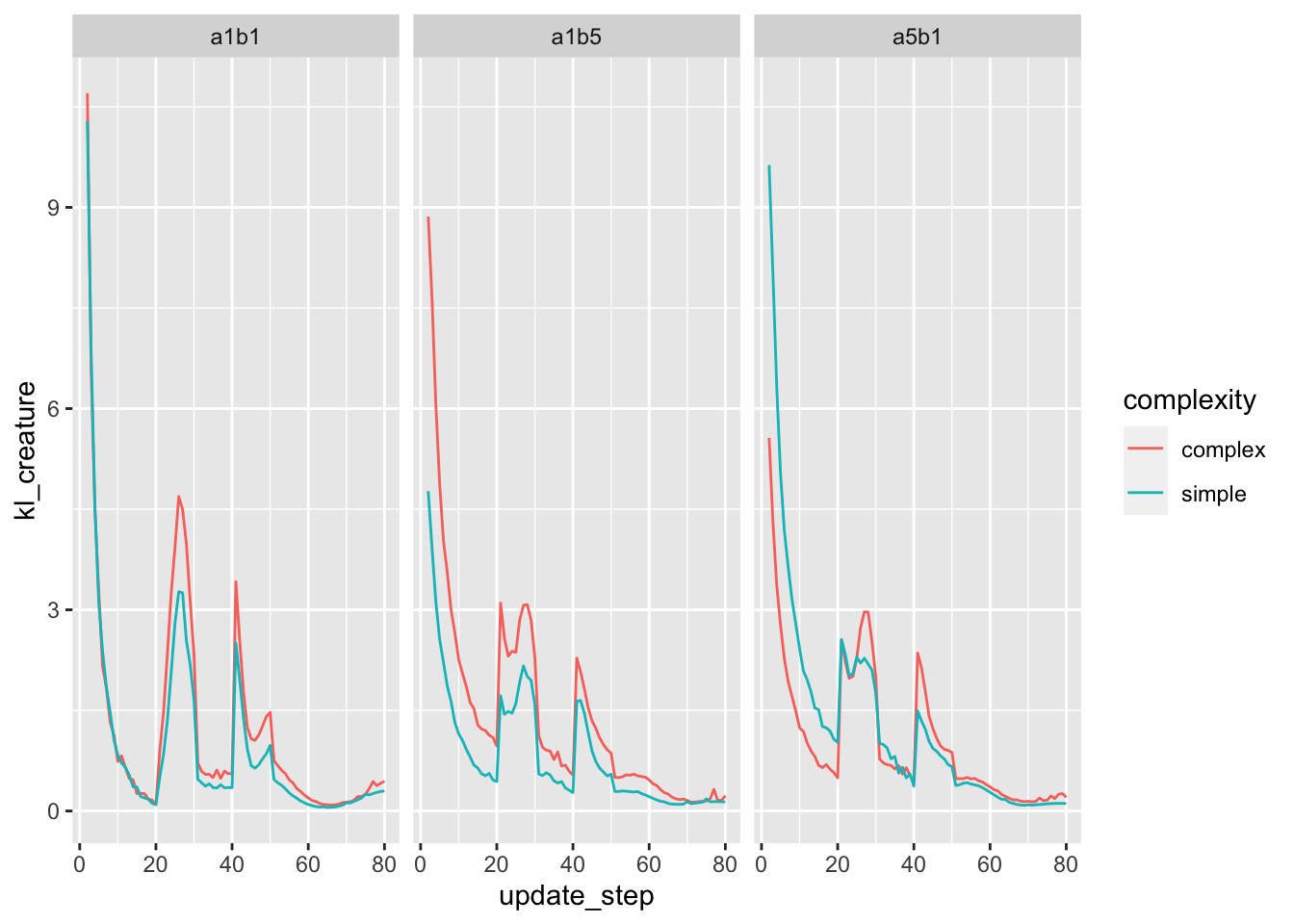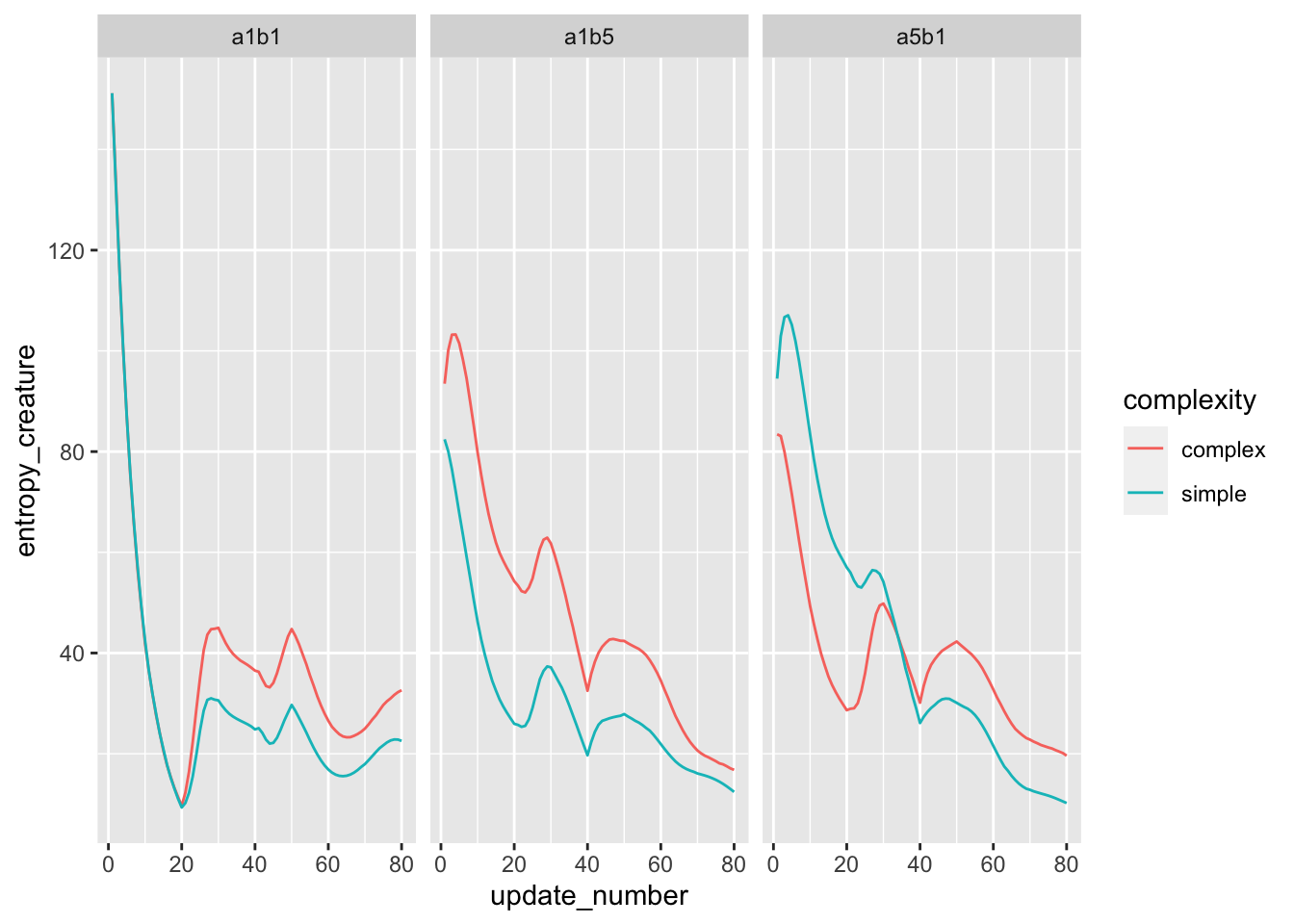library(tidyverse)
## ── Attaching packages ─────────────────────────────────────── tidyverse 1.3.0 ──
## ✓ ggplot2 3.3.3     ✓ purrr   0.3.4
## ✓ tibble  3.1.0     ✓ dplyr   1.0.5
## ✓ tidyr   1.1.1     ✓ stringr 1.4.0
## ✓ readr   1.3.1     ✓ forcats 0.4.0
## ── Conflicts ────────────────────────────────────────── tidyverse_conflicts() ──
## x dplyr::lag()    masks stats::lag()
library(here)
## here() starts at /Users/caoanjie/Desktop/projects/looking_time/adult_analysis
source(here("adult_modeling/scripts/get_entropy.R"))
source(here("adult_modeling/scripts/get_surprise.R"))

Stimuli seqeuence: - 8 trial, deviants at 3rd and 5th trial
- total feature 100; simple feature n = 30 , complex feature = 80; deviants have 20% different features - all feature theta = 0.8; all non-feature theta = 0.2

observation sequence: - each trial has 10 observations - epsilon = 0.02

noisy observation update: - grid theta and grid epsilon: seq(0.1, 1, 0.2) - alpha_prior = 1; beta_prior = 1 - alpha_epsilon = 1; beta_epsilon = 10

s_a1b1 <- readRDS(here("adult_modeling/m_res/obs_1_sequential_update.rds"))

c_a5b1 <- readRDS(here("adult_modeling/m_res/obs_2_a5b1_sequential_update.rds"))

# KL divergence

here we calculate the expected information gain (EIG) for a new observation $$z_{t+1}$$ at time point $$t$$

$D_{KL}(p(\theta|z_{i+1}) || p(\theta|z_{i})) = \sum_{\theta \in [\theta_{1}, \theta_{2}...\theta_{d}]} p(\theta|z_{i+1}) log\frac{p(\theta|z_{i+1})}{p(\theta|z_{i}))}$

So for each observation at time point $$z_{t+1}$$, we summed over all the possible grid $\theta$ value.

kl_s_a1b1 <- readRDS(here("adult_modeling/m_res/obs_1_sequential_update_kl.rds")) %>%
mutate(complexity = "simple",
params = "a1b1")

mutate(complexity = "complex",
params = "a1b1")

mutate(complexity = "simple",
params = "a1b5")

mutate(complexity = "complex",
params = "a1b5")

mutate(complexity = "simple",
params = "a5b1")

mutate(complexity = "complex",
params = "a5b1")#needs rerun

kl_all <- bind_rows(kl_s_a1b1,
kl_c_a1b1,
kl_s_a1b5,
kl_c_a1b5,
kl_s_a5b1,
kl_c_a5b1)

kl_all %>%
filter(complexity == "complex")
## # A tibble: 23,700 x 7
##         kl update_step feature_index trial_num trial_observation_num complexity
##      <dbl>       <dbl>         <dbl>     <int>                 <dbl> <chr>
##  1 0.0998            2             1         1                    10 complex
##  2 0.0600            3             1         1                    10 complex
##  3 0.0387            4             1         1                    10 complex
##  4 0.0264            5             1         1                    10 complex
##  5 0.0188            6             1         1                    10 complex
##  6 0.0138            7             1         1                    10 complex
##  7 0.0104            8             1         1                    10 complex
##  8 0.00791           9             1         1                    10 complex
##  9 0.00612          10             1         1                    10 complex
## 10 0.00477          11             1         2                    10 complex
## # … with 23,690 more rows, and 1 more variable: params <chr>
kl_all %>%
group_by(update_step, complexity, params) %>%
summarise(kl_creature = sum(kl)) %>%
ggplot(aes(x = update_step,
y = kl_creature,
color = complexity)) +
geom_line() +
facet_wrap(~params)
## summarise() has grouped output by 'update_step', 'complexity'. You can override using the .groups argument.# entropy

following the notation given in:http://todd.gureckislab.org/2021/05/05/negative-information

The prior is so the entropy at timestep 0 is $g_0 = H(\theta) = -\sum_{\theta}p(\theta)logp(\theta)$

and after i update the entropy will become $g_{i}(z_i) = H(\theta|z_i) = -\sum_{\theta}p(\theta|z_i)logp(\theta|z_i)$

translate the computation to code looks something like this:

get_entropy_for_feature_one_update <- function(lps){
-sum(lps * exp(lps))
}

Poli uses negative entropy as a measurement for predictability, so here we will do something similar.

s_a1b1_e <- get_entropy_for_creature_udpates(s_a1b1) %>%
mutate(complexity = "simple",
params = "a1b1")
c_a1b1_e <- get_entropy_for_creature_udpates(c_a1b1) %>%
mutate(complexity = "complex",
params = "a1b1")

s_a1b5_e <- get_entropy_for_creature_udpates(s_a1b5) %>%
mutate(complexity = "simple",
params = "a1b5")

c_a1b5_e <- get_entropy_for_creature_udpates(c_a1b5)%>%
mutate(complexity = "complex",
params = "a1b5")

s_a5b1_e <- get_entropy_for_creature_udpates(s_a5b1)%>%
mutate(complexity = "simple",
params = "a5b1")
c_a5b1_e <- get_entropy_for_creature_udpates(c_a5b1)%>%
mutate(complexity = "complex",
params = "a5b1")

e_all <- bind_rows(s_a1b1_e, c_a1b1_e,
s_a1b5_e, c_a1b5_e,
s_a5b1_e, c_a5b1_e) 

a little bit weird, shouldn’t this be upside down? this is the entropy not the negative entropy aka the surprise

e_all %>%
group_by(update_number, complexity, params) %>%
summarise(entropy_creature = sum(e)) %>%
ggplot(aes(x = update_number,
y = entropy_creature,
color = complexity)) +
geom_line() +
facet_wrap(~params)
## summarise() has grouped output by 'update_number', 'complexity'. You can override using the .groups argument.# surprisal

this is what got me confused the most. currently calculating as: surprisal at observation $$z_{t}$$ just the weighted average of the surprisal for each value of theta, and take the average of those surprisals weighed by p(theta = this_particular_value_of_theta|z) (so doing an weighted average over $p(\theta|z_{t}))$?

this is looks exactly like the entropy so i must be doing something wrong?? unless they are technically the same thing?

s_a1b1_s <- get_surprise_for_creature_updates(s_a1b1) %>%
mutate(complexity = "simple",
params = "a1b1")
mutate(complexity = "complex",
params = "a1b1")

mutate(complexity = "simple",
params = "a1b5")

mutate(complexity = "complex",
params = "a1b5")

mutate(complexity = "simple",
params = "a5b1")
mutate(complexity = "complex",
params = "a5b1")

s_all <- bind_rows(s_a1b1_s, c_a1b1_s,
s_a1b5_s, c_a1b5_s,
s_a5b1_s, c_a5b1_s) 
s_all %>%
group_by(update_number, complexity, params) %>%
summarise(surprise_creature = sum(surprise)) %>%
ggplot(aes(x = update_number,
y = surprise_creature,
color = complexity)) +
geom_line() +
facet_wrap(~params)
## summarise() has grouped output by 'update_number', 'complexity'. You can override using the .groups argument.